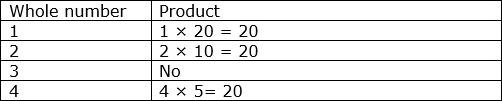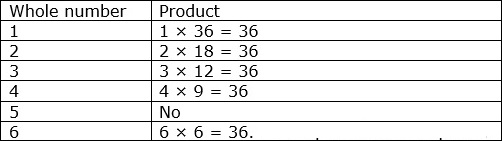# Factors

Factors are the numbers we multiply to get another number. For example, if we multiply 3 and 5, we get 15. We say, 3 and 5 are factors of 15.

Alternatively, factors of a number are those numbers which divide that number without leaving any remainder.

For example, 1, 2, 3, 4, 6, and 12 are factors of 12 as all of them divide it evenly.

### The largest and smallest factors of a number

• The largest factor of any number is the number itself and the smallest factor is 1.
• 1 is the factor of every number.
• So, for example, the largest and smallest factors of 12 are 12 and 1.

Find all the factors of 20

### Solution

Step 1:

We go through the whole numbers as shown below in table.

To find factors, we try to make products equal to 20.Step 2:

We stop when get to a factor that repeats.

Step 3:

So all factors of 20 are 1, 2, 4, 5, 10, and 20

Find all the factors of 36

### Solution

Step 1:

We go through the whole numbers as shown.

To find all factors, we try to make products equal to 36.Step 2:

We stop when get to a factor that repeats.

Step 3:

So all the factors of 36 are 1, 2, 3, 4, 6, 9, 12, 18 and 36